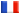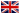# Séminaire de Cryptographie

## Aurore Guillevic### Computing individual discrete logarithms in non-prime finite fields

This talk is about computing discrete logarithms in non-prime finite fields. These fields arise in pairing-based cryptography. In this setting, the pairing-friendly curve is defined over GF(q) and the pairing takes its values in an extension GF(q^k), where k is the embedding degree. Fr example, GF(p^2) is the embedding field of supersingular elliptic curves in large characteristic; GF(p^3), GF(p^4), GF(p^6) are the embedding fields of MNT curves, and GF(p^12) is the embedding field of the well-known Barreto-Naehrig curves. In small characteristic, GF(2^(4*n)), GF(3^(6*m)) are considered.

To compute discrete logarithms in these fields, one uses the Number Field Sieve algorithm (NFS) in large characteristic (e.g. GF(p^2)), the NFS-High-Degree variant (NFS-HD) in medium characteristic (e.g. GF(p^12)) and the Quasi Polynomial-time Algorithm (QPA) in small characteristic when applicable. These algorithms are made of four steps: polynomial selection, relation collection, linear algebra modulo the prime order of the target group and finally, individual logarithm computation.

All these finite fields are extensions, hence have subfields. We use their structure to speed-up the individual discrete logarithm computation. We obtain an important speed-up in practice and the best case is when the embedding degree k is even. We will illustrate the improvements with the practical case of GF(p^4) with p^4 of 400 bits (120 decimal digits).